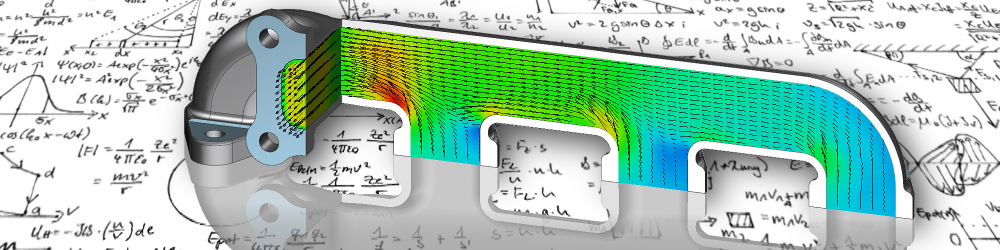•• 1

# Turbulent flow

Turbulence is a widespread, almost omnipresent phenomenon in fluid mechanics. This is understood as the apparently random and disordered, vortex-like movement patterns within a flowing field, which change continuously and thereby vary in size and shape. This continuous variation of the flow direction or fluctuation around a main flow direction results in increased mixing, which not only takes place at the material or molecular level, but also takes into account the state variables such as energy and impulse. This increases the diffusion coefficient.

## DNS: Direct Numerical Simulation

The most exact solution of a turbulent flow can be achieved by a direct numerical simulation, abbreviated DNS. However, since the vortices are not stable and each cascade dissolves again and again into several small vortices, very fine networks and very small time steps are necessary to detect the actual flow. The minimum requirements for this result from the Kolmogorov scales.

For this reason, numerical flow investigations using DNS are extremely complex and can only be carried out for very simple geometries and with a moderate Reynolds number for the foreseeable future. In normal engineering practice, therefore, it has almost no significance.

## RANS: Reynolds Averaged Navier-Stokes equations

In many cases it is not necessary for the technical use of numerical flow simulation to consider the details of each individual spatial and temporal change in the flow quantities, but rather only their effect on the total flow. For this purpose, the state variables are divided into their mean and fluctuation values over time. By inserting them into the basic equations, the Reynolds-averaged Navier-Stokes equations or RANS equations are obtained. This includes unknown variables for the fluctuating components in the turbulent flow. Turbulence models are used to describe these as a function of the mean flow.

Over the years, various models have been established for this purpose, which can be divided into two groups. The first group consists of the vortex viscosity models, which are based on the assumption of a direction-independent, i.e. isotropic turbulence behavior. These include

• the K-ε model,
• the K-ω model,
• the SST model as well as
• the model according to Spalart-Allmaras.

The second group consists of the Reynold stress models, in which the simplifying hypothesis of the directional independence of turbulence, which is particularly inaccurate in the wall area of the flow, is no longer maintained. Well-known representatives of this group are

• the τ-ε model and
• the τ-ω model.

For industrial problems, numerical flow simulation is almost exclusively based on the RANS equations. The quality of the results depends, among other factors, on the validity limits of the turbulence model used. Despite their known uncertainty, the preference in practice lies on the eddy viscosity models due to the lower numerical effort.

## LES: Large Eddy Simulation

The Large Eddy Simulation, or LES for short, is a mixture of the two preceding methods. Here larger vertebral structures are solved directly and only the smaller ones are described with a model. Since small structure turbulence behaves differently from coarse structure turbulence, a simpler, special fine structure turbulence model can be formed.

Despite this simplification, the computational effort for a large eddy simulation remains very high, although the quality of the results is also close to that of direct numerical simulation.Warmesberg 24, 9450 Altstätten, Switzerland

+41 79 471 1612